# Calculate weighted average in Excel PivotTable in 2 ways

Here are a few methods on how to calculate the weighted average in Excel PivotTable or DAX. It is not so simple as a weighted average by using calculation in an Excel worksheet. The good news is that you can use that method to validate your results.

Here are 2 ways to create this calculation, considering that the weighted average should work by every Excel PivotTable group.

## Weighted average calculation in Excel PivotTable with helper column

The helper column is necessary to calculate multiplication with weight for the calculation.

1. Multiply the column that you want to use in the weighted average calculation with the weight. That is the same that you would use in the weighted average calculation in an Excel worksheet.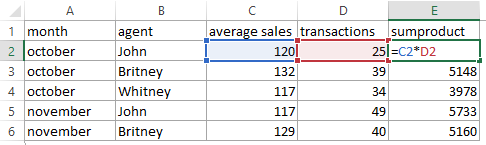2. Use this data in the creation of PivotTable.

3. Add the necessary field to the PivotTable row grouping. When something is added to PivotTable, go to the tab PivotTable Analyze and then Field, Items & Sets -> Calculated Field.

4. Create weighted average calculation in Excel PivotTable by dividing helper column by weight.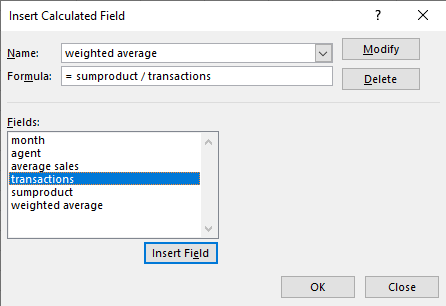## Weighted average without a helper column by using DAX, works also in Power BI

Sometimes it is necessary to get the weighted average in Excel PivotTable without a helper column. Use Power Pivot capabilities that are basically DAX usage in Excel.

#### Add existing PivotTable to the data model

Firstly, in Excel, data should be added to the data model. It is possible to do that by creating a PivotTable. If you have an existing PivotTable, then here is how to add that to the data model.

Select PivotTable and in the PivotTable fields section, click More Tables and say yes to the pop-up question.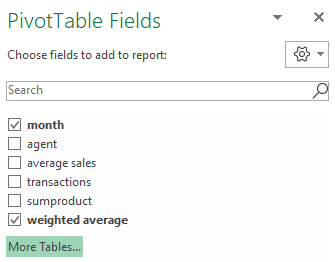That will recreate the same PivotTable that you have.

#### Weighted average without a helper column

1. In the Excel Data tab and open the PowerPivot window.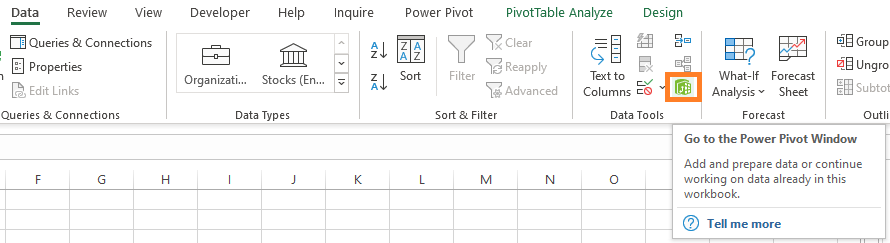2. Create a DAX measure to calculate the weighted average in the calculation area (section at the bottom). Here is more about SUMX.

`weighted average:= SUMX(Range, [average sales] * [transactions]) / SUM([transactions])`

3. Weighted average calculation measure should be available in the PivotTable fields section.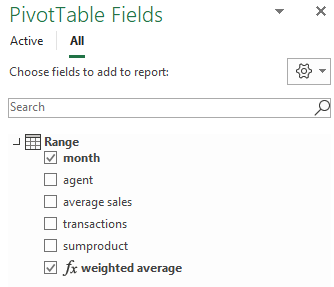## Weighted average in Power BI

Similarly, use the same weighted average DAX calculation in Power BI.

`weighted average = SUMX(Range, [average sales] * [transactions]) / SUM([transactions])`